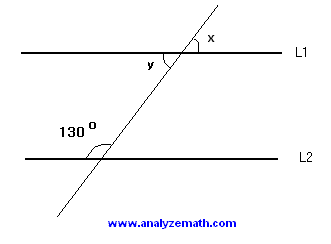#Free Mathematics Tutorials# GMAT Geometry Problems with Solutions and Explanations Sample 2

Solutions with detailed explanations to problems in sample 2.

## Solution to Question 1

Let y be the vertical angle to x. Since L1 and L2 are parallel, angle y and the angle with measure 130� are supplementary. Hence.

y + 130 = 180 or y = 180 - 130 = 50�
x and y are vertical angles and therefore have equal measures
x = 50�

## Solution to Question 2

The sum of all 3 angles of any triangle is equal to 180 degrees. Hence
(4x + 10) + (4x + 5) + (3x) = 180
Group like terms and solve for x
11x = 165 or x = 15

## Solution to Question 3

The area A of the given triangle may be calculated using the two altitutdes as follows
A = (1/2)(AN)(BC) or A = (1/2)(BM)(AC)
Hence
(1/2)(AN)(BC) = (1/2)(BM)(AC)
Multiply both sides by 2 and substitute known lengths
8 * 10 = MB * 12
Multiply both sides by 2 and substitute known lengths
BM = 80 / 12 = 6.7 (approximated to one decimal place)

## Solution to Question 4

Since BA and BC have equal lengths, then the triangle is isosceles and the interior angles at A and C have equal measues which may be calculated as follows
A + C + 40 = 180 or 2A = 140 or A = 70�
The interior angle at A and angle x are supplementary. Hence
70 + x = 180 or x = 110�

## Solution to Question 5

The two legs of a right isosceles triangle have equal lengths; let x be one of these lengths. The area A of the triangle is given by
A = (1/2) x * x = (1/2)x
2
We now use Pythagorean theorem to find x as follows
x
2 + x2 = 242
Simplify
2 x
2 = 576
x
2 = 288
We now calculate the area A as follows
A = (1/2)x
2 = (1/2) 288 = 144

## Solution to Question 6

Let us express the total area as the area of the upper and lower triangles with commom base AC
(1/2)(20)(AC) + (1/2)(28)(AC) = 240
Multiply all terms by 2, simplify and solve for AC
(20)(AC) + (28)(AC) = 480
48 AC = 480
AC = 480 / 48 = 10

## Solution to Question 7

Let x be the side of the square. The area is equal to x2. Hence
x
2 = 144 , solve for x, x = 12
The diameter d of the circle is equal to the length of the diagonal of the square. Using Pythagora's theorem, we obtain
x
2 + x2 = d2
Solve for d
144 + 144 = d
2
d = 12 √2
The radius r of the circle is equal to d/2
r = 12 √2 / 2 = 6 √2
The area A of the circle is given by
A = Pi r
2 = 72 Pi

## Solution to Question 8

Any two consecutive angle of a parallelogram are supplementary. Hence
(2x + 10) + (x + 20) = 180
Solve for x
3x + 30 = 180
x = 50
We now evaluate M and N as follows
M = 2x + 10 = 2(50) + 10 = 110�
N = x + 20 = 50 + 20 = 70�

## Solution to Question 9

Let L and W be the length and width of the rectangle. Using the formula of the perimeter, we write
2L + 2W = 90
W is given; hence
2L + 2(10) = 90
Solve for L
2L = 70 , L = 35
The area A of the rectangle is given by
A = L * W = 35 * 10 = 350

## Solution to Question 10

The area of a square of side x is x2 and the area of a rectangle of width 2x and length 3x is (2 x)(3 x) = 6 x2. The ratio of the area of the square to the area of a rectangle is given by
x
2 / (6 x2) = 1 / 6

## More References and Links to More Math Practice Tests

Free Practice for GAMT Math tests
Free Compass Math tests Practice
Free Practice for SAT, ACT Math tests
Free GRE Quantitative for Practice
Free AP Calculus Questions (AB and BC) with Answers